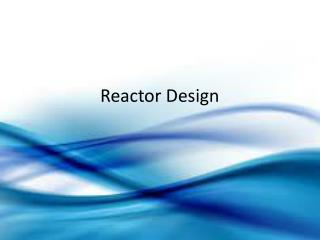# Reactor Design - PowerPoint PPT PresentationDownload PresentationReactor Design

Reactor Design
Download Presentation## Reactor Design

- - - - - - - - - - - - - - - - - - - - - - - - - - - E N D - - - - - - - - - - - - - - - - - - - - - - - - - - -
##### Presentation Transcript

1. Reactor Design

2. Batch Reactor

3. Batch reactors are closed systems that operate under unsteady-state conditions. • Batch reactors are used when only a small amount of product is desired. For this reason batch reactors are favored when a process is still in the testing phase, or when the desired product is expensive.

4. The movie to the left shows the basic operation of a batch reactor. The reactants are placed into the reactor and then allowed to react. The products and unreacted reactants are then removed and the process is repeated.

5. What is the total time of each batch?

6. Batch Reactor Design Equation If “A” is the limiting reactant

7. Sheet 4

8. The liquid phase reaction: With known kinetics r = k CA (k = 5 x 10-3 sec-1) is to be affected in a batch reactor. The concentration of A in feed equals 2 kmole/m3. • How long would it take to achieve 30% conversion of A? • What fractional conversion can be achieved after an operation time of 100 sec? • If the feed concentration of A is increased, would the corresponding fractional conversion of A after 100 sec increase or decrease with respect to (ii)?

9. Butyl acetate was formed in a batch-operated reactor at 100ºC with conc. H2SO4 as a homogeneous catalyst. The original feed contained 4.97 molesbutanol per mole of acetic acid. The following rate equation was found to correlate the data: r = k CA2 where CA is the concentration of acetic acid in gmole/mlit and r is the rate of reaction in gmole of acetic acid consumed/mlit. min. and k = 17.4 cm3/gmole.min. Assuming the density of the mixture is constant and equal to 0.75 gm/cm3. Calculate the time required to reach a conversion of 50%.

10. The liquid phase reaction: With the following rate equation: r = k CA CB , k = 0.05 m3/kmole.sec. The reaction takes place in a batch reactor. The initial concentrations of A and B are: CAf = CBf= 2 kmole/m3 • What time is necessary to attain 50% conversion? • If the feed concentration of B is 3 kmole/m3, what time is necessary to attain 50% conversion of A?

11. polymath

12. Resolve the 3 problems using POLYMATH and compare the results with the previous results.